Related Articles
How to export Pandas DataFrame to a CSV file?
• Last Updated : 10 Jul, 2020

Let us see how to export a Pandas DataFrame to a CSV file. We will be using the `to_csv()` function to save a DataFrame as a CSV file.

## DataFrame.to_csv()

Syntax : to_csv(parameters)
Parameters :

• path_or_buf : File path or object, if None is provided the result is returned as a string.
• sep : String of length 1. Field delimiter for the output file.
• na_rep : Missing data representation.
• float_format : Format string for floating point numbers.
• columns : Columns to write.
• header : If a list of strings is given it is assumed to be aliases for the column names.
• index : Write row names (index).
• index_label : Column label for index column(s) if desired. If None is given, and header and index are True, then the index names are used.
• mode : Python write mode, default ‘w’.
• encoding : A string representing the encoding to use in the output file.
• compression : Compression mode among the following possible values: {‘infer’, ‘gzip’, ‘bz2’, ‘zip’, ‘xz’, None}.
• quoting : Defaults to csv.QUOTE_MINIMAL.
• quotechar : String of length 1. Character used to quote fields.
• line_terminator : The newline character or character sequence to use in the output file.
• chunksize : Rows to write at a time.
• date_format : Format string for datetime objects.
• doublequote : Control quoting of quotechar inside a field.
• escapechar : String of length 1. Character used to escape sep and quotechar when appropriate.
• decimal : Character recognized as decimal separator. E.g. use ‘,’ for European data.

Returns : None or str

Example 1 :

 `# importing the module ` `import` `pandas as pd ` ` `  `# creating the DataFrame ` `my_df ``=` `{``'Name'``: [``'Rutuja'``, ``'Anuja'``],  ` `         ``'ID'``: [``1``, ``2``],  ` `         ``'Age'``: [``20``, ``19``]} ` `df ``=` `pd.DataFrame(my_df) ` ` `  `# displaying the DataFrame ` `print``(``'DataFrame:\n'``, df) ` `  `  `# saving the DataFrame as a CSV file ` `gfg_csv_data ``=` `df.to_csv(``'GfG.csv'``, index ``=` `True``) ` `print``(``'\nCSV String:\n'``, gfg_csv_data) `

Output :
Before executing the code: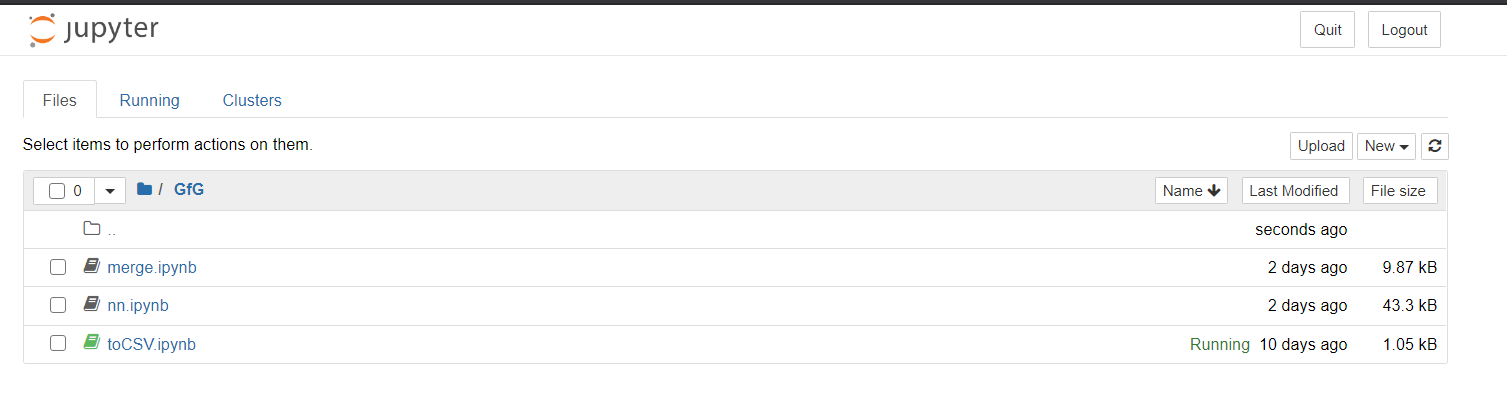After executing the code: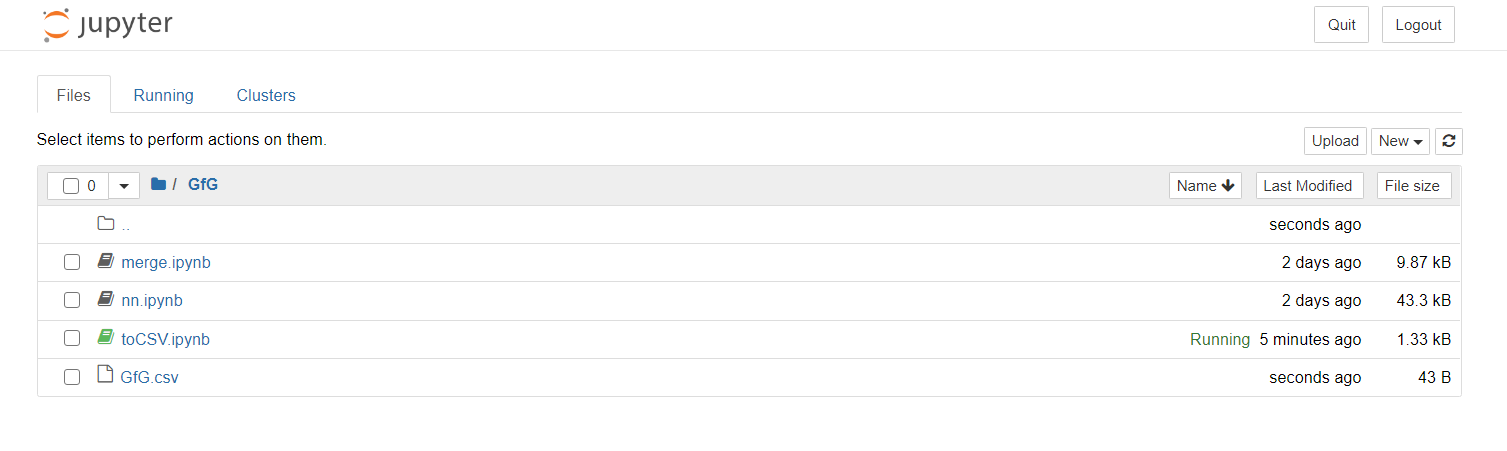We can clearly see the .csv file created.

Also, the output of the above code includes the index, as follows.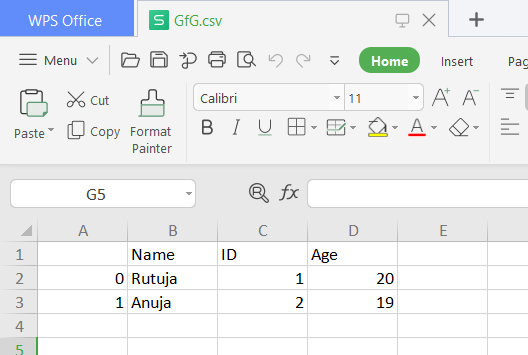Example 2 : Converting to a CSV file without the index. If we wish not to include the index, then in the `index `parameter assign the value `False`.

 `# importing the module ` `import` `pandas as pd ` ` `  `# creating the DataFrame ` `my_df ``=` `{``'Name'``: [``'Rutuja'``, ``'Anuja'``],  ` `         ``'ID'``: [``1``, ``2``],  ` `         ``'Age'``: [``20``, ``19``]} ` `df ``=` `pd.DataFrame(my_df) ` ` `  `# displaying the DataFrame ` `print``(``'DataFrame:\n'``, df) ` `  `  `# saving the DataFrame as a CSV file ` `gfg_csv_data ``=` `df.to_csv(``'GfG.csv'``, index ``=` `False``) ` `print``(``'\nCSV String:\n'``, gfg_csv_data) `

Output: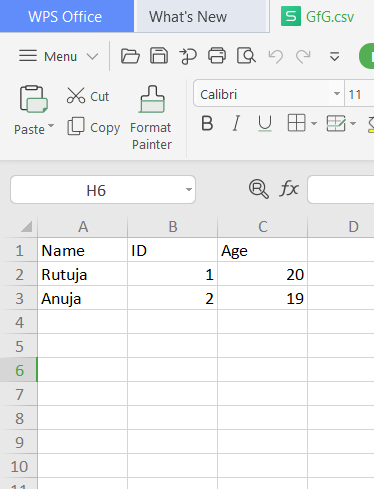Example 3 : Converting to a CSV file without the header of the rows. If we wish not to include the header, then in the `header`parameter assign the value `False`.

 `# importing the module ` `import` `pandas as pd ` ` `  `# creating the DataFrame ` `my_df ``=` `{``'Name'``: [``'Rutuja'``, ``'Anuja'``],  ` `         ``'ID'``: [``1``, ``2``],  ` `         ``'Age'``: [``20``, ``19``]} ` `df ``=` `pd.DataFrame(my_df) ` ` `  `# displaying the DataFrame ` `print``(``'DataFrame:\n'``, df) ` `  `  `# saving the DataFrame as a CSV file ` `gfg_csv_data ``=` `df.to_csv(``'GfG.csv'``, header ``=` `False``) ` `print``(``'\nCSV String:\n'``, gfg_csv_data) `

Output: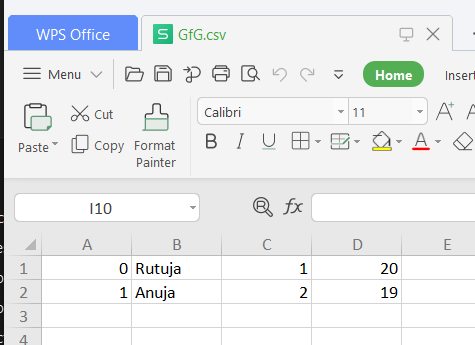Attention geek! Strengthen your foundations with the Python Programming Foundation Course and learn the basics.

To begin with, your interview preparations Enhance your Data Structures concepts with the Python DS Course.

My Personal Notes arrow_drop_up
Recommended Articles
Page :